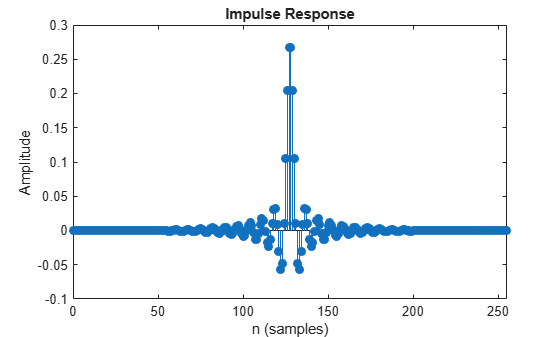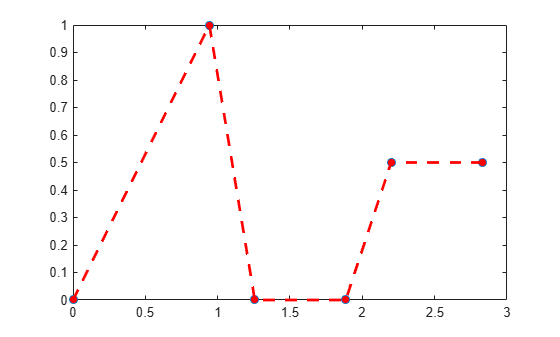# firls

Least-square linear-phase FIR filter design

## Syntax

``b = firls(n,f,a)``
``b = firls(n,f,a,w)``
``b = firls(n,f,a,ftype)``
``b = firls(n,f,a,w,ftype)``

## Description

example

````b = firls(n,f,a)` returns row vector `b` containing the `n+1` coefficients of the order `n` FIR filter. This filter has frequency-amplitude characteristics approximately matching those given by vectors, `f` and `a`. ```
````b = firls(n,f,a,w)` uses the weights in vector `w`, to weigh the error. ```

example

````b = firls(n,f,a,ftype)` specifies a filter type where `ftype` is:`'hilbert'``'differentiator'` ```
````b = firls(n,f,a,w,ftype)` uses the weights in vector `w` to weigh the error. It also specifies a filter type where `ftype` is:`'hilbert'``'differentiator'` ```

## Examples

collapse all

The following illustrates how to design a lowpass filter of order 225 with transition band.

Create the frequency and amplitude vectors, `f` and `a`.

`f = [0 0.25 0.3 1]`
```f = 1×4 0 0.2500 0.3000 1.0000 ```
`a = [1 1 0 0]`
```a = 1×4 1 1 0 0 ```

Use `firls` to obtain the `n+1` coefficients of the order `n` lowpass FIR filter.

`b = firls(255,f,a);`

Show the impulse response of the filter

`fvtool(b,'impulse')`The following shows how to design a 24th-order anti-symmetric filter with piecewise linear passbands, and plot the desired and actual amplitude responses.

Create the frequency and amplitude vectors, `f` and `a`.

```f = [0 0.3 0.4 0.6 0.7 0.9]; a = [0 1 0 0 0.5 0.5];```

Use `firls` to obtain the 25 coefficients of the filter.

`b = firls(24,f,a,'hilbert');`

Plot the ideal amplitude response along with the transition regions.

```plot(f.*pi,a,'o','markerfacecolor',[1 0 0]); hold on; plot(f.*pi,a,'r--','linewidth',2);```Use `freqz` to obtain the frequency response of the designed filter and plot the magnitude response of the filter.

```[H,F] = freqz(b,1); plot(F,abs(H)); set(gca,'xlim',[0 pi]) legend('Filter Specification','Transition Regions','Magnitude Response')```## Input Arguments

collapse all

Order of the filter, specified as an integer scalar. For odd orders, the frequency response at the Nyquist frequency is necessarily 0. For this reason, `firls` always uses an even filter order for configurations with a passband at the Nyquist frequency. If you specify an odd-valued `n`, `firls` increments it by 1.

Example: `8`

Data Types: `int8` | `int16` | `int32` | `int64`

Pairs of frequency points, specified as a vector of values ranging between 0 and 1, where 1 corresponds to the Nyquist frequency. The frequencies must be in increasing order, and duplicate frequency points are allowed. You can use duplicate frequency points to design filters exactly like those returned by the `fir1` and `fir2` functions with a rectangular (`rectwin`) window.

`f` and `a` are the same length. This length must be an even number.

Example: `[0 0.3 0.4 1]`

Data Types: `double` | `single`

Amplitude values of the function at each frequency point, specified as a vector of the same length as `f`. This length must be an even number.

The desired amplitude at frequencies between pairs of points (f(k), f(k+1)) for k odd, is the line segment connecting the points (f(k), a(k)) and (f(k+1), a(k+1)).

The desired amplitude at frequencies between pairs of points (f(k), f(k+1)) for k even is unspecified. These are transition or “don't care” regions.

Example: `[1 1 0 0]`

Data Types: `double` | `single`

Weights to weigh the fit for each frequency band, specified as a vector of length half the length of `f` and `a`, so there is exactly one weight per band. `w` indicates how much emphasis to put on minimizing the integral squared error in each band, relative to the other bands.

Example: `[0.5 1]`

Data Types: `double` | `single`

Filter type, specified as either `'hilbert'` or `'differentiator'`.

Example: ‘hilbert’

Data Types: `char`

## Output Arguments

collapse all

Filter coefficients, returned as a numeric vector of `n+1` values, where `n` is the filter order.

`b = firls(n,f,a)` designs a linear-phase filter of type I (`n` odd) and type II (`n`) . The output coefficients, or “taps,” in `b` obey the relation:

b(k) = b(n+2-k), k = 1, ... , n + 1

`b = firls(n,f,a,'hilbert')` designs a linear-phase filter with odd symmetry (type III and type IV). The output coefficients, or “taps,” in `b` obey the relation:

b(k) = –b(n+2-k), k = 1, ... , n + 1

`b = firls(n,f,a,'differentiator')` designs type III and type IV filters, using a special weighting technique. For nonzero amplitude bands, the integrated squared error has a weight of (1/f)2. This weighting causes the error at low frequencies to be much smaller than at high frequencies. For FIR differentiators, which have an amplitude characteristic proportional to frequency, the filters minimize the relative integrated squared error. This value is the integral of the square of the ratio of the error to the desired amplitude.

collapse all

### Diagnostics

Error and warning messages

One of the following diagnostic messages is displayed when an incorrect argument is used:

```F must be even length. F and A must be equal lengths. Requires symmetry to be 'hilbert' or 'differentiator'. Requires one weight per band. Frequencies in F must be nondecreasing. Frequencies in F must be in range [0,1]. ```

A more serious warning message is

```Warning: Matrix is close to singular or badly scaled. ```

This tends to happen when the product of the filter length and transition width grows large. In this case, the filter coefficients b might not represent the desired filter. You can check the filter by looking at its frequency response.

## Algorithms

`firls` designs a linear-phase FIR filter. This filter minimizes the weighted, integrated squared error between an ideal piecewise linear function and the magnitude response of the filter over a set of desired frequency bands.

Reference  describes the theoretical approach behind `firls`. The function solves a system of linear equations involving an inner product matrix of size roughly `n/2` using the MATLAB® \ operator.

This function designs type I, II, III, and IV linear-phase filters. Type I and II are the defaults for n even and odd respectively. The `'hilbert'` and `'differentiator'` flags produce type III (n even) and IV (n odd) filters. The various filter types have different symmetries and constraints on their frequency responses (see  for details).

Linear Phase Filter TypeFilter OrderSymmetry of CoefficientsResponse H(f), f = 0Response H(f), f = 1 (Nyquist)

Type I

Even

b(k) = b(n+2-k), k=1,..., n+1

No restriction

No restriction

Type II

Odd

b(k) = b(n+2-k), k=1,..., n+1

No restriction

H(1) = 0

Type III

Even

b(k) = –b(n+2-k), k=1,..., n+1

H(0) = 0

H(1) = 0

Type IV

Odd

b(k) = –b(n+2-k), k=1,..., n+1

H(0) = 0

No restriction

 Parks, T.W., and C.S. Burrus, Digital Filter Design, John Wiley & Sons, 1987, pp. 54-83.

 Oppenheim, A.V., and R.W. Schafer, Discrete-Time Signal Processing, Prentice-Hall, 1989, pp. 256-266.